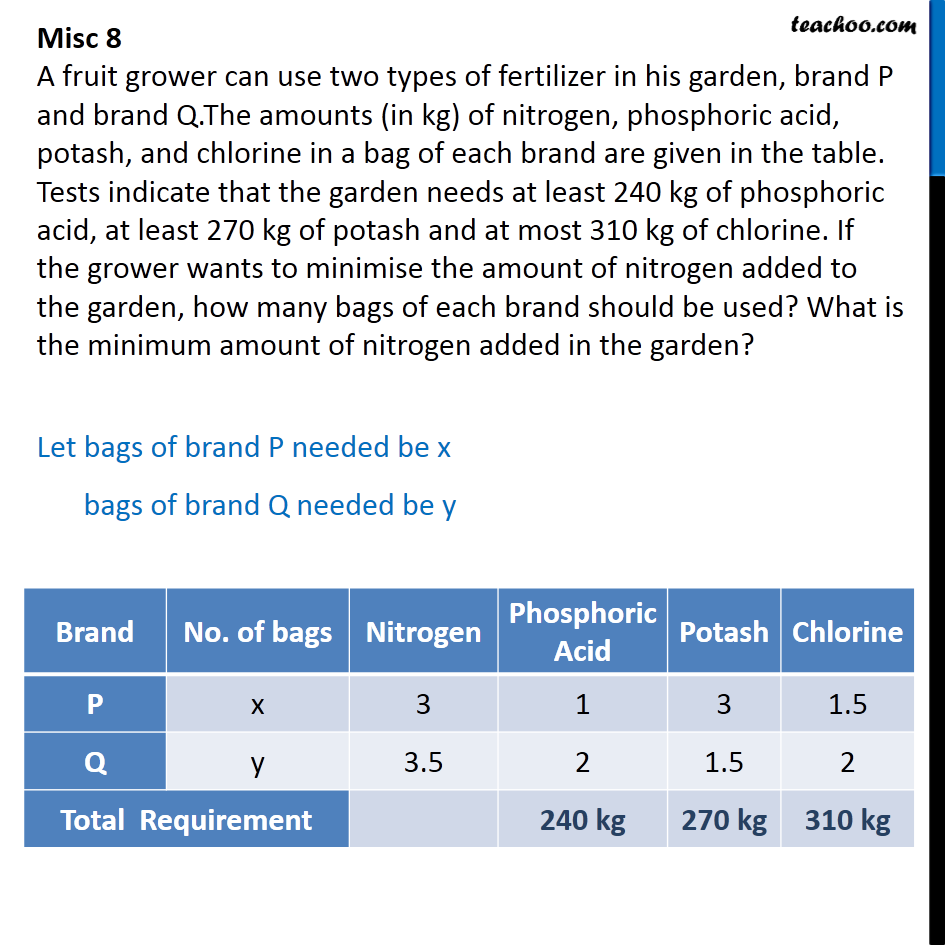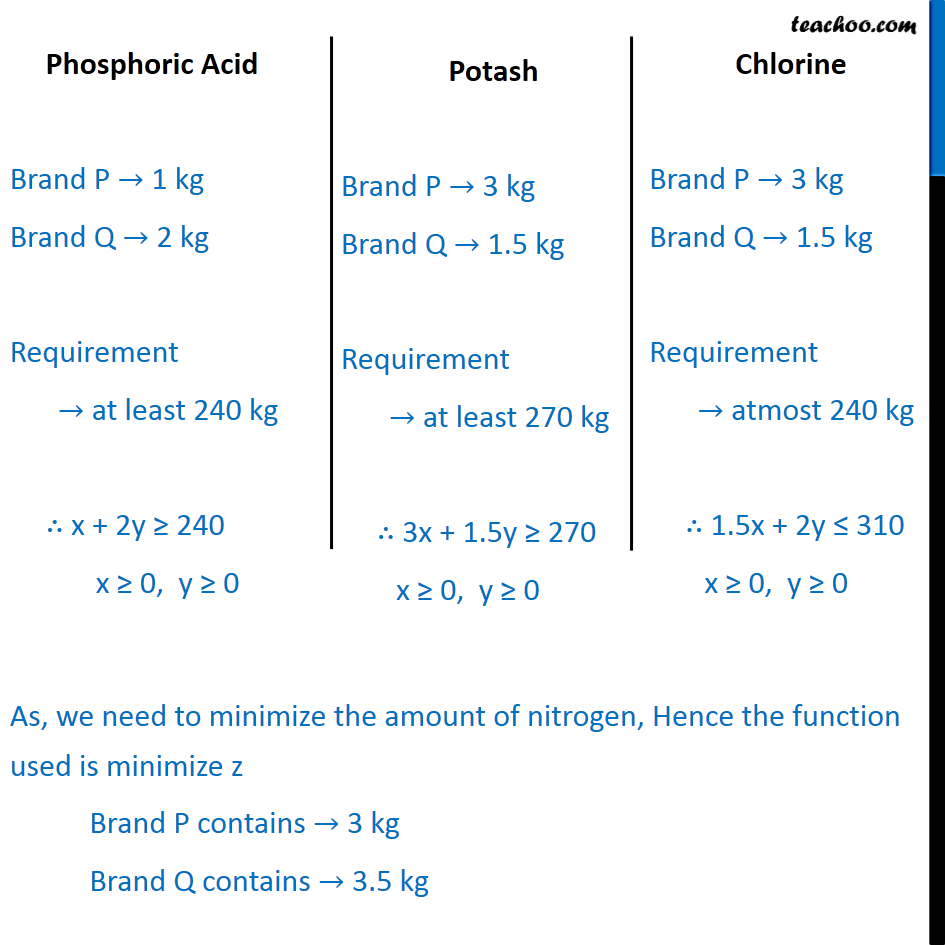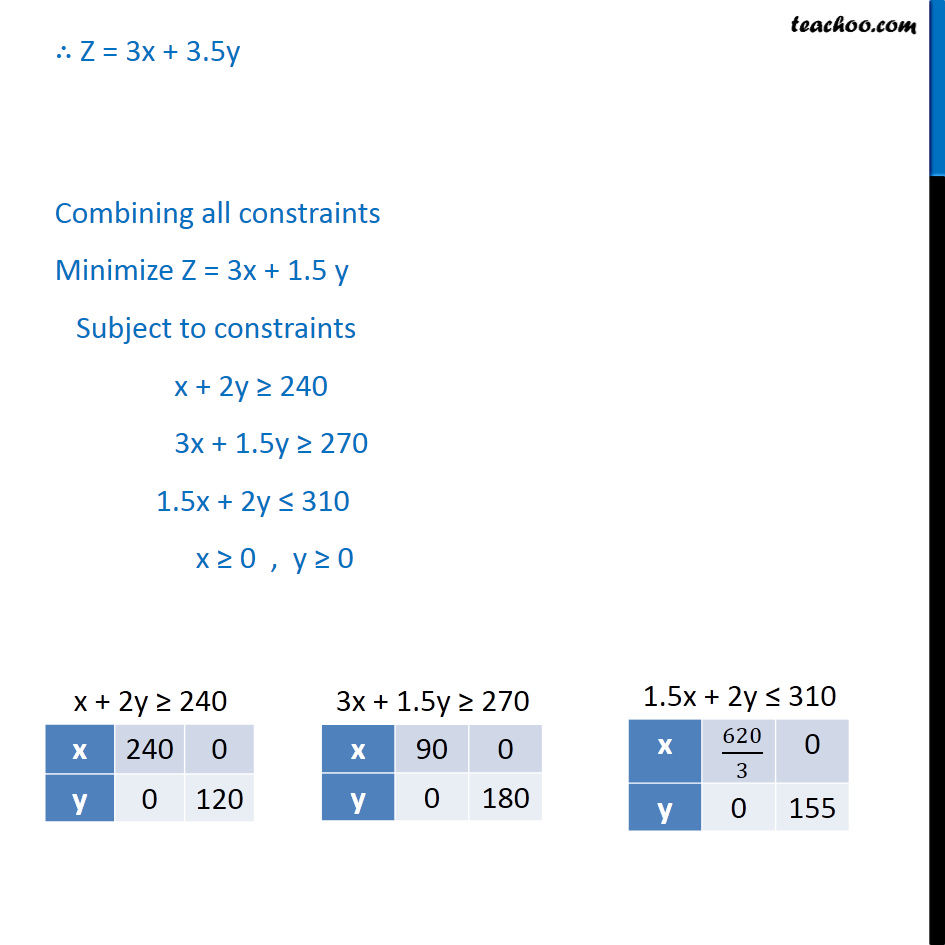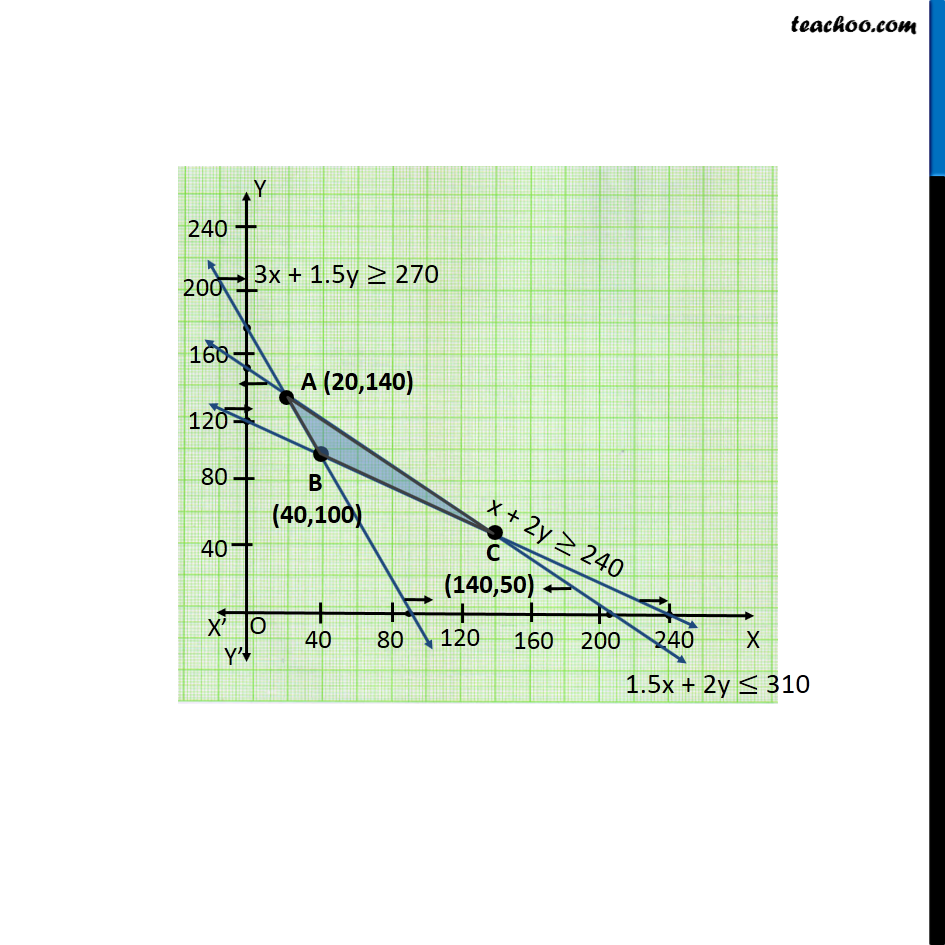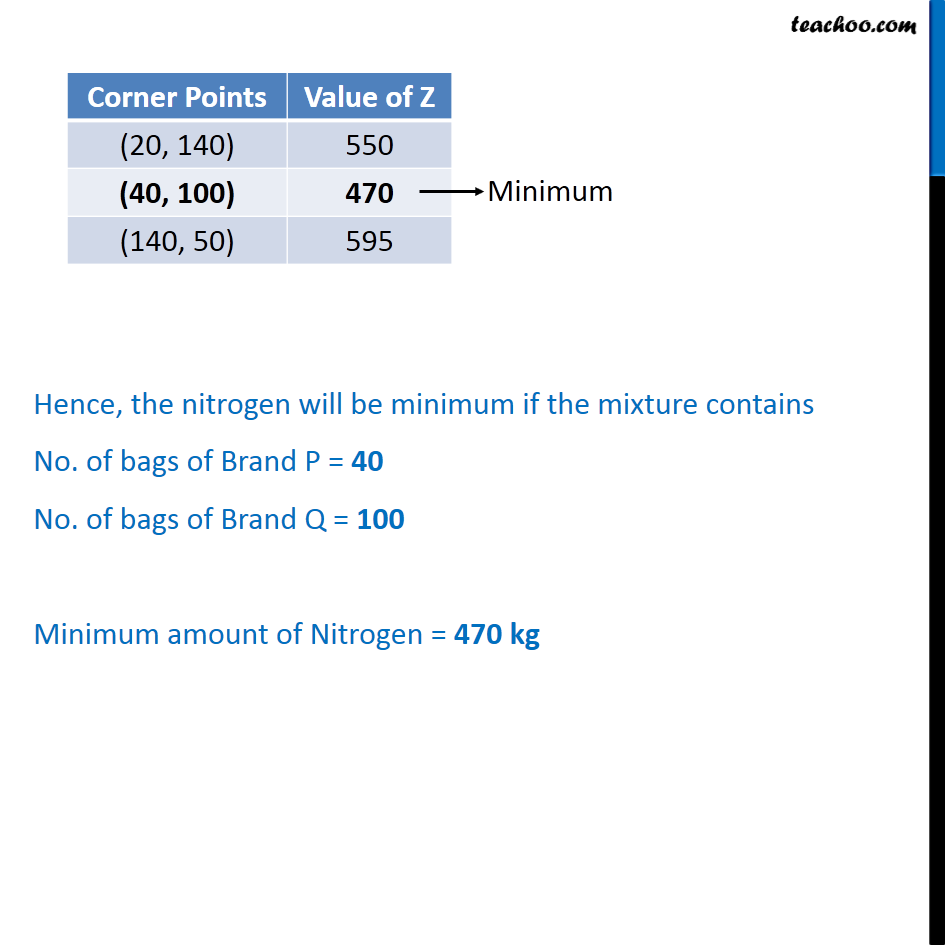1. Chapter 12 Class 12 Linear Programming
2. Serial order wise
3. Miscellaneous

Transcript

Misc 8 A fruit grower can use two types of fertilizer in his garden, brand P and brand Q.The amounts (in kg) of nitrogen, phosphoric acid, potash, and chlorine in a bag of each brand are given in the table. Tests indicate that the garden needs at least 240 kg of phosphoric acid, at least 270 kg of potash and at most 310 kg of chlorine. If the grower wants to minimise the amount of nitrogen added to the garden, how many bags of each brand should be used? What is the minimum amount of nitrogen added in the garden? Let bags of brand P needed be x bags of brand Q needed be y As, we need to minimize the amount of nitrogen, Hence the function used is minimize z Brand P contains 3 kg Brand Q contains 3.5 kg Z = 3x + 3.5y Combining all constraints Minimize Z = 3x + 1.5 y Subject to constraints x + 2y 240 3x + 1.5y 270 1.5x + 2y 310 x 0 , y 0 Hence, the nitrogen will be minimum if the mixture contains No. of bags of Brand P = 40 No. of bags of Brand Q = 100 Minimum amount of Nitrogen = 470 kg

Miscellaneous